# Chapter 6 Sampling and Estimation Statistical Sampling Sampling

• Slides: 36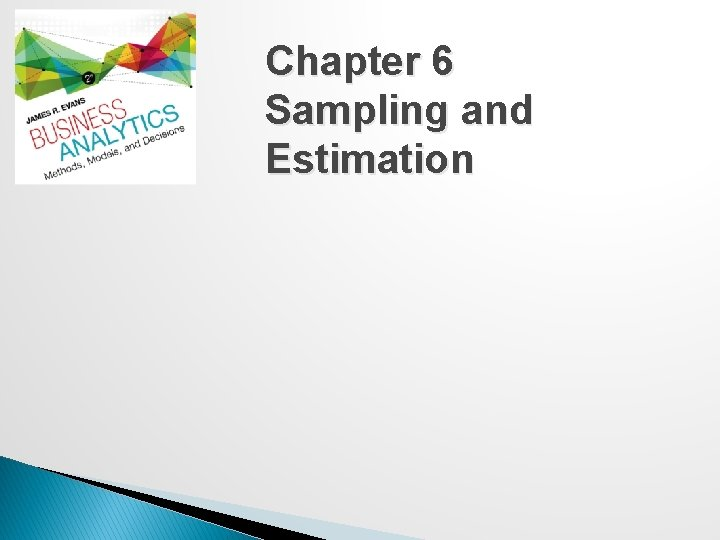Chapter 6 Sampling and EstimationStatistical Sampling � Sampling is the foundation of statistical analysis. � Sampling plan - a description of the approach that is used to obtain samples from a population prior to any data collection activity. � A sampling plan states: - its objectives - target population - population frame (the list from which the sample is selected) - operational procedures for collecting data - statistical tools for data analysisExample 6. 1: A Sampling Plan for a Market Research Study A company wants to understand how golfers might respond to a membership program that provides discounts at golf courses. ◦ Objective - estimate the proportion of golfers who would join the program ◦ Target population - golfers over 25 years old ◦ Population frame - golfers who purchased equipment at particular stores ◦ Operational procedures - e-mail link to survey or direct-mail questionnaire ◦ Statistical tools - Pivot. Tables to summarize data by demographic groups and estimate likelihood of joining the programSampling Methods � Subjective Methods �Judgment sampling – expert judgment is used to select the sample �Convenience sampling – samples are selected based on the ease with which the data can be collected � Probabilistic Sampling �Simple random sampling involves selecting items from a population so that every subset of a given size has an equal chance of being selected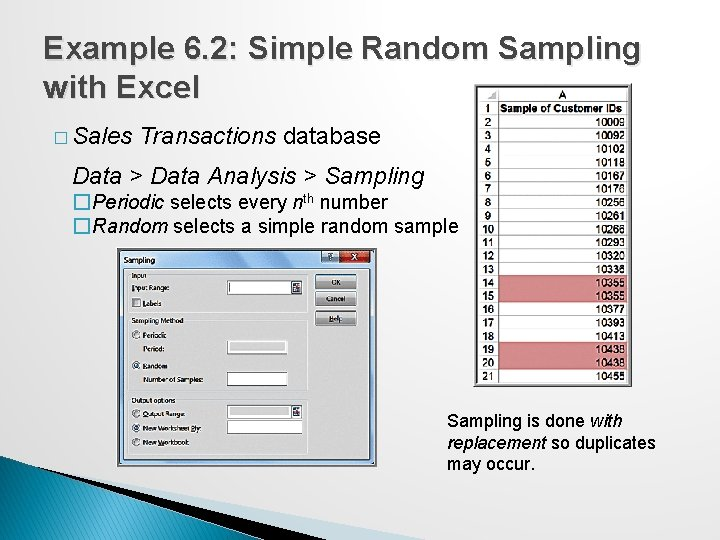Example 6. 2: Simple Random Sampling with Excel � Sales Transactions database Data > Data Analysis > Sampling �Periodic selects every nth number �Random selects a simple random sample Sampling is done with replacement so duplicates may occur.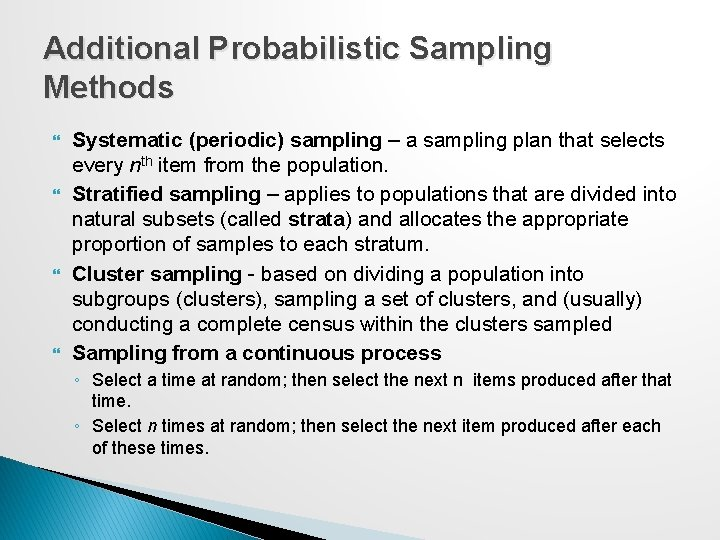Additional Probabilistic Sampling Methods Systematic (periodic) sampling – a sampling plan that selects every nth item from the population. Stratified sampling – applies to populations that are divided into natural subsets (called strata) and allocates the appropriate proportion of samples to each stratum. Cluster sampling - based on dividing a population into subgroups (clusters), sampling a set of clusters, and (usually) conducting a complete census within the clusters sampled Sampling from a continuous process ◦ Select a time at random; then select the next n items produced after that time. ◦ Select n times at random; then select the next item produced after each of these times.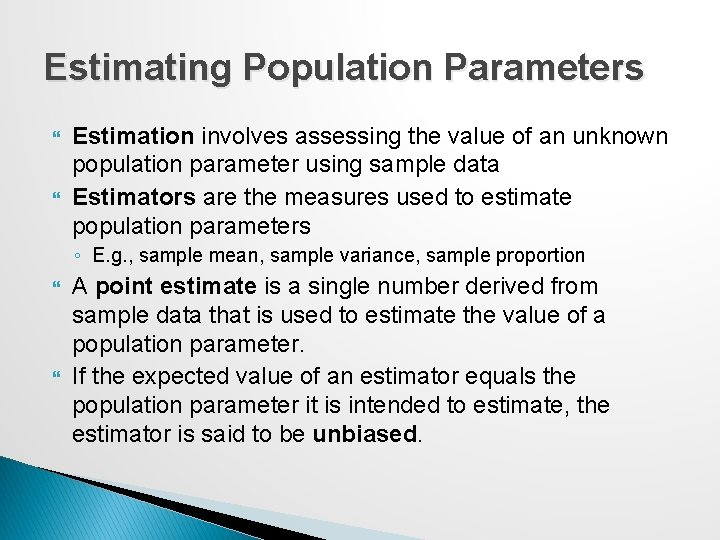Estimating Population Parameters Estimation involves assessing the value of an unknown population parameter using sample data Estimators are the measures used to estimate population parameters ◦ E. g. , sample mean, sample variance, sample proportion A point estimate is a single number derived from sample data that is used to estimate the value of a population parameter. If the expected value of an estimator equals the population parameter it is intended to estimate, the estimator is said to be unbiased.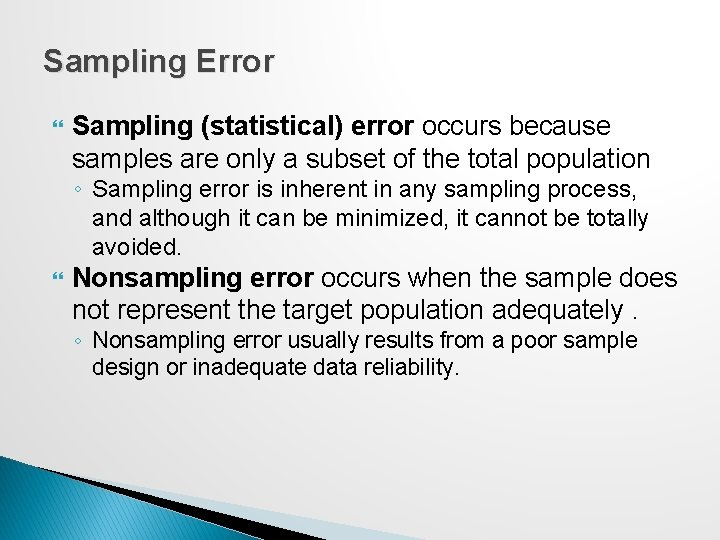Sampling Error Sampling (statistical) error occurs because samples are only a subset of the total population ◦ Sampling error is inherent in any sampling process, and although it can be minimized, it cannot be totally avoided. Nonsampling error occurs when the sample does not represent the target population adequately. ◦ Nonsampling error usually results from a poor sample design or inadequate data reliability.Example 6. 3: A Sampling Experiment A population is uniformly distributed between 0 and 10. ◦ Mean = (0 + 10)/2 = 5 ◦ Variance = (10 − 0)2/12 = 8. 333 Experiment: ◦ ◦ ◦ Generate 25 samples of size 10 from this population. Compute the mean of each sample. Prepare a histogram of the 250 observations, Prepare a histogram of the 25 sample means. Repeat for larger sample sizes and draw comparative conclusions.Example 6. 3: Experiment Results Note that the average of all the sample means is quite close the true population mean of 5. 0.Example 6. 3: Other Sample Sizes Repeat the sampling experiment for samples of size 25, 100, and 500 As the sample size increases, the average of the sample means are all still close to the expected value of 5; however, the standard deviation of the sample means becomes smaller, meaning that the means of samples are clustered closer together around the true expected value. The distributions become normal.Example 6. 4: Estimating Sampling Error Using the Empirical Rules � Using the empirical rule for 3 standard deviations away from the mean, ~99. 7% of sample means should be between: [2. 55, 7. 45] for n = 10 [3. 65, 6. 35] for n = 25 [4. 09, 5. 91] for n = 100 [4. 76, 5. 24] for n = 500 As the sample size increases, the sampling error decreases.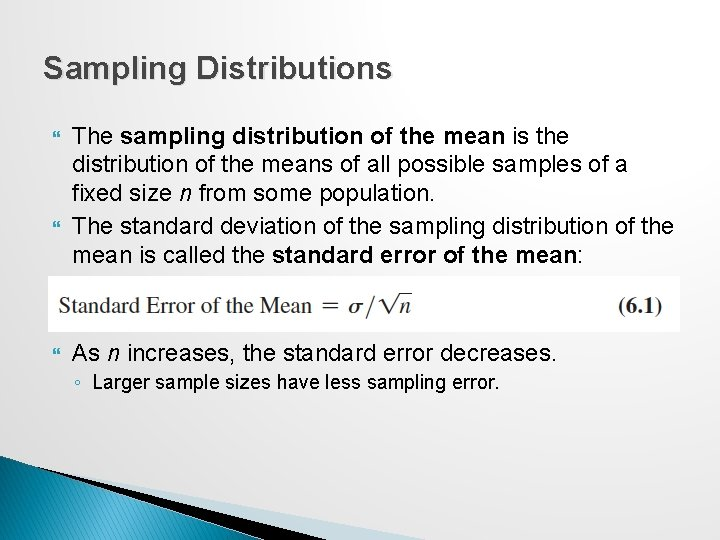Sampling Distributions The sampling distribution of the mean is the distribution of the means of all possible samples of a fixed size n from some population. The standard deviation of the sampling distribution of the mean is called the standard error of the mean: As n increases, the standard error decreases. ◦ Larger sample sizes have less sampling error.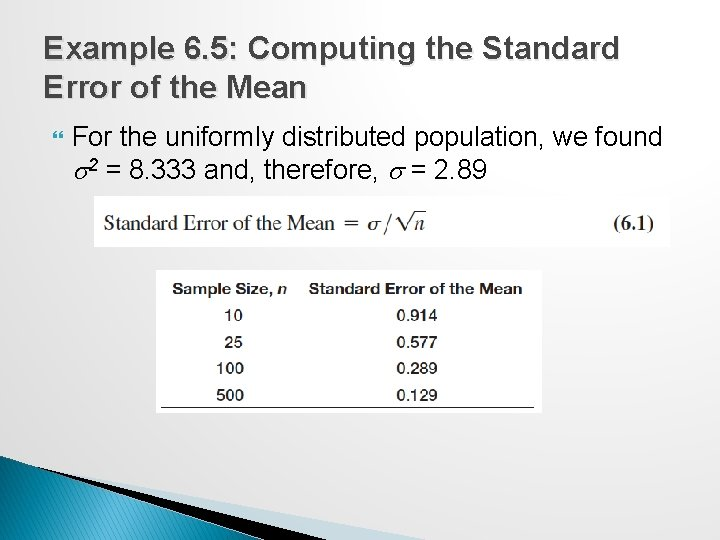Example 6. 5: Computing the Standard Error of the Mean For the uniformly distributed population, we found s 2 = 8. 333 and, therefore, s = 2. 89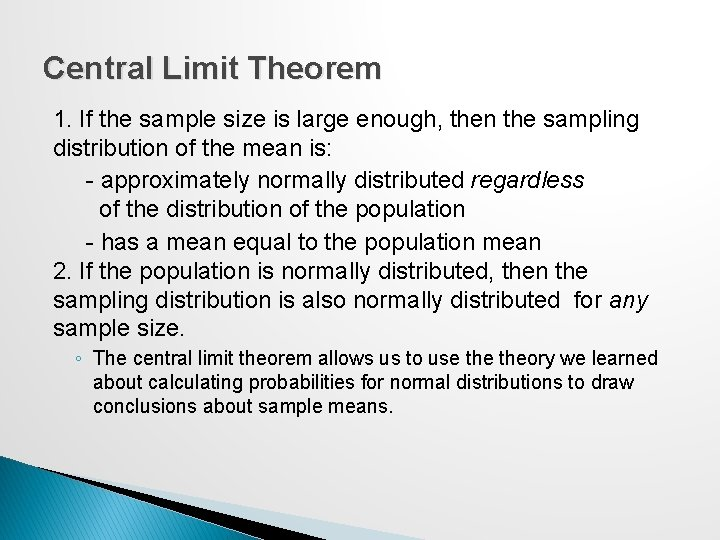Central Limit Theorem 1. If the sample size is large enough, then the sampling distribution of the mean is: - approximately normally distributed regardless of the distribution of the population - has a mean equal to the population mean 2. If the population is normally distributed, then the sampling distribution is also normally distributed for any sample size. ◦ The central limit theorem allows us to use theory we learned about calculating probabilities for normal distributions to draw conclusions about sample means.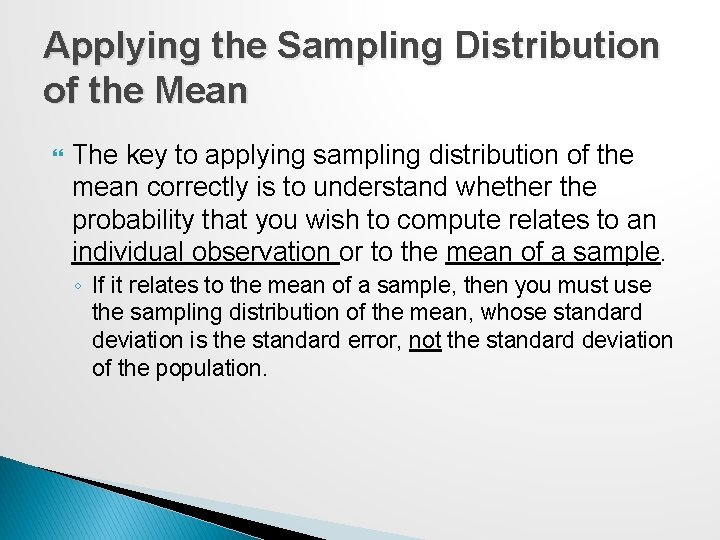Applying the Sampling Distribution of the Mean The key to applying sampling distribution of the mean correctly is to understand whether the probability that you wish to compute relates to an individual observation or to the mean of a sample. ◦ If it relates to the mean of a sample, then you must use the sampling distribution of the mean, whose standard deviation is the standard error, not the standard deviation of the population.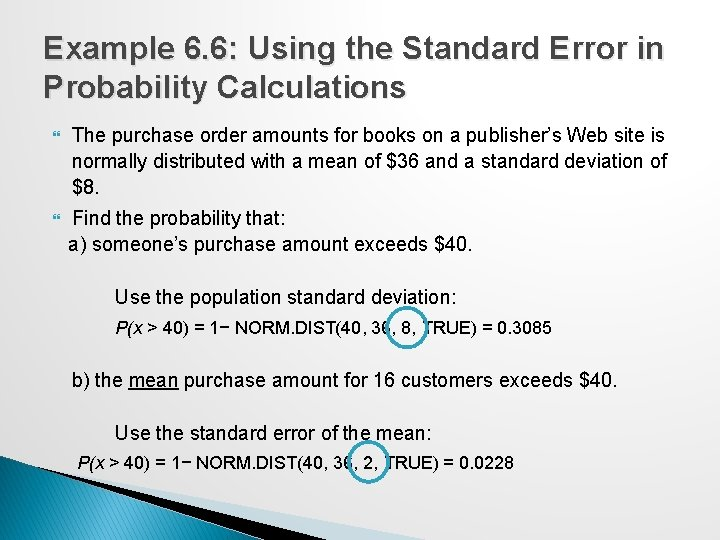Example 6. 6: Using the Standard Error in Probability Calculations The purchase order amounts for books on a publisher’s Web site is normally distributed with a mean of \$36 and a standard deviation of \$8. Find the probability that: a) someone’s purchase amount exceeds \$40. Use the population standard deviation: P(x > 40) = 1− NORM. DIST(40, 36, 8, TRUE) = 0. 3085 b) the mean purchase amount for 16 customers exceeds \$40. Use the standard error of the mean: P(x > 40) = 1− NORM. DIST(40, 36, 2, TRUE) = 0. 0228Interval Estimates An interval estimate provides a range for a population characteristic based on a sample. ◦ Intervals specify a range of plausible values for the characteristic of interest and a way of assessing “how plausible” they are. In general, a 100(1 - a)% probability interval is any interval [A, B] such that the probability of falling between A and B is 1 - a. ◦ Probability intervals are often centered on the mean or median. ◦ Example: in a normal distribution, the mean plus or minus 1 standard deviation describes an approximate 68% probability interval around the mean.Example 6. 7: Interval Estimates in the News A Gallup poll might report that 56% of voters support a certain candidate with a margin of error of ± 3%. ◦ We would have a lot of confidence that the candidate would win since the interval estimate is [53%, 59%] Suppose the poll reported a 52% level of support with a ± 4% margin of error. ◦ We would be less confident in predicting a win for the candidate since the interval estimate is [48%, 56%].Confidence Intervals A confidence interval is a range of values between which the value of the population parameter is believed to be, along with a probability that the interval correctly estimates the true (unknown) population parameter. ◦ This probability is called the level of confidence, denoted by 1 a, where a is a number between 0 and 1. ◦ The level of confidence is usually expressed as a percent; common values are 90%, 95%, or 99%. For a 95% confidence interval, if we chose 100 different samples, leading to 100 different interval estimates, we would expect that 95% of them would contain the true population mean.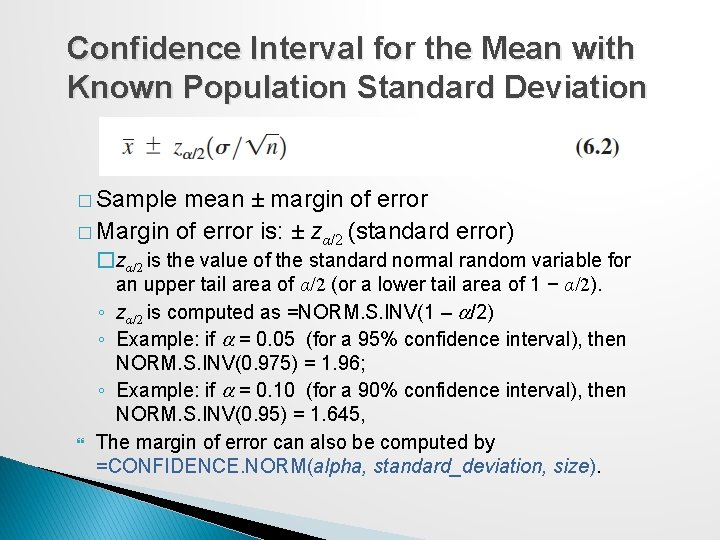Confidence Interval for the Mean with Known Population Standard Deviation � Sample mean ± margin of error � Margin of error is: ± zα/2 (standard error) �zα/2 is the value of the standard normal random variable for an upper tail area of α/2 (or a lower tail area of 1 − α/2). ◦ zα/2 is computed as =NORM. S. INV(1 – a/2) ◦ Example: if a = 0. 05 (for a 95% confidence interval), then NORM. S. INV(0. 975) = 1. 96; ◦ Example: if a = 0. 10 (for a 90% confidence interval), then NORM. S. INV(0. 95) = 1. 645, The margin of error can also be computed by =CONFIDENCE. NORM(alpha, standard_deviation, size).Example 6. 8: Computing a Confidence Interval with a Known Standard Deviation A production process fills bottles of liquid detergent. The standard deviation in filling volumes is constant at 15 mls. A sample of 25 bottles revealed a mean filling volume of 796 mls. A 95% confidence interval estimate of the mean filling volume for the population isExcel Workbook for Confidence Intervals The worksheet Population Mean Sigma Known in the Excel workbook Confidence Intervals computes this interval using the CONFIDENCE. NORM functionConfidence Interval Properties As the level of confidence, 1 - a, decreases, za/2 decreases, and the confidence interval becomes narrower. ◦ For example, a 90% confidence interval will be narrower than a 95% confidence interval. Similarly, a 99% confidence interval will be wider than a 95% confidence interval. Essentially, you must trade off a higher level of accuracy with the risk that the confidence interval does not contain the true mean. ◦ To reduce the risk, you should consider increasing the sample size.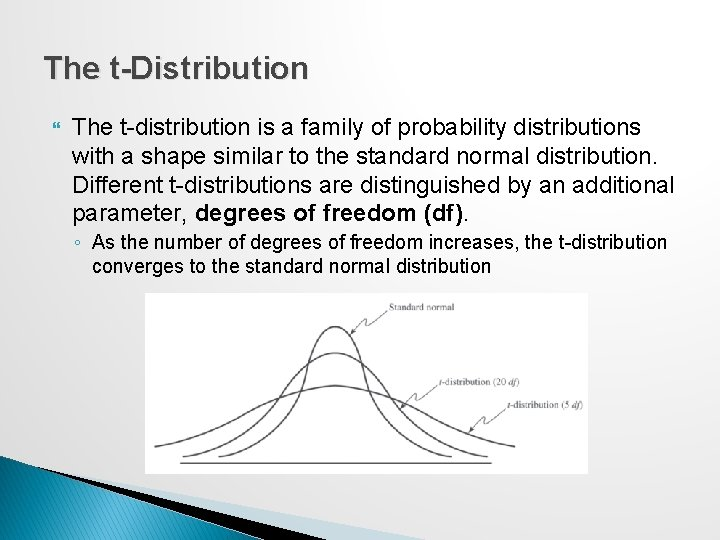The t-Distribution The t-distribution is a family of probability distributions with a shape similar to the standard normal distribution. Different t-distributions are distinguished by an additional parameter, degrees of freedom (df). ◦ As the number of degrees of freedom increases, the t-distribution converges to the standard normal distributionConfidence Interval for the Mean with Unknown Population Standard Deviation where tα/2 is the value of the t-distribution with df = n − 1 for an upper tail area of α/2. � t values are found in Table 2 of Appendix A or with the Excel function T. INV(1 – a/2, n – 1). � The Excel function =CONFIDENCE. T(alpha, standard_deviation, size) can be used to compute the margin of error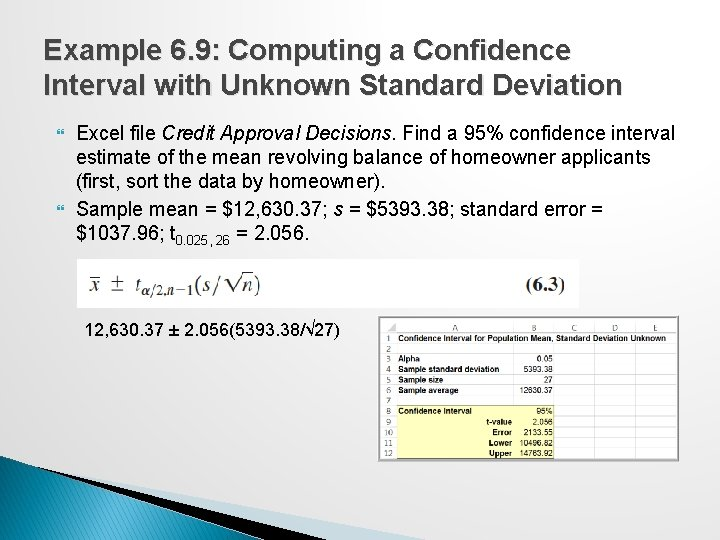Example 6. 9: Computing a Confidence Interval with Unknown Standard Deviation Excel file Credit Approval Decisions. Find a 95% confidence interval estimate of the mean revolving balance of homeowner applicants (first, sort the data by homeowner). Sample mean = \$12, 630. 37; s = \$5393. 38; standard error = \$1037. 96; t 0. 025, 26 = 2. 056. 12, 630. 37 ± 2. 056(5393. 38/√ 27)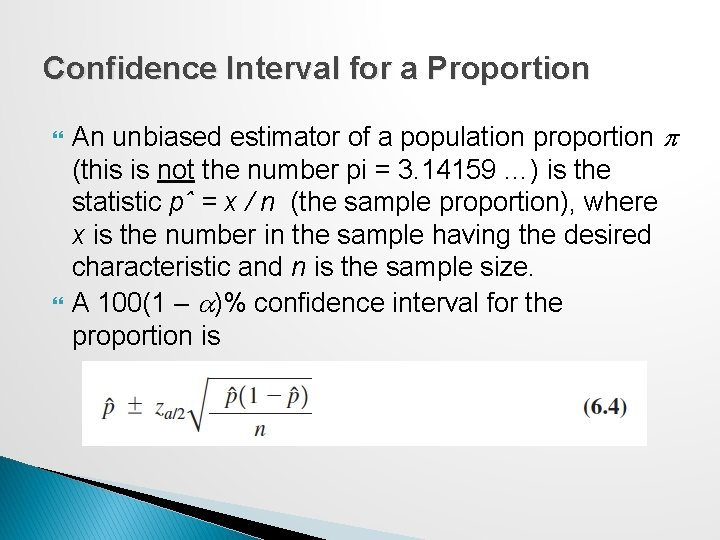Confidence Interval for a Proportion An unbiased estimator of a population proportion p (this is not the number pi = 3. 14159 …) is the statistic pˆ = x / n (the sample proportion), where x is the number in the sample having the desired characteristic and n is the sample size. A 100(1 – a)% confidence interval for the proportion isExample 6. 10: Computing a Confidence Interval for a Proportion Excel file Insurance Survey. We are interested in the proportion of individuals who would be willing to pay a lower premium for a higher deductible for their health insurance. ◦ Sample proportion = 6/24 = 0. 25. Confidence interval: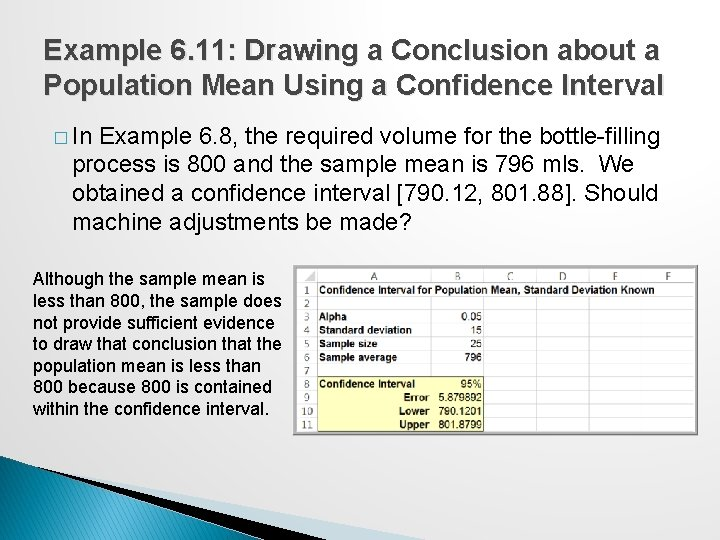Example 6. 11: Drawing a Conclusion about a Population Mean Using a Confidence Interval � In Example 6. 8, the required volume for the bottle-filling process is 800 and the sample mean is 796 mls. We obtained a confidence interval [790. 12, 801. 88]. Should machine adjustments be made? Although the sample mean is less than 800, the sample does not provide sufficient evidence to draw that conclusion that the population mean is less than 800 because 800 is contained within the confidence interval.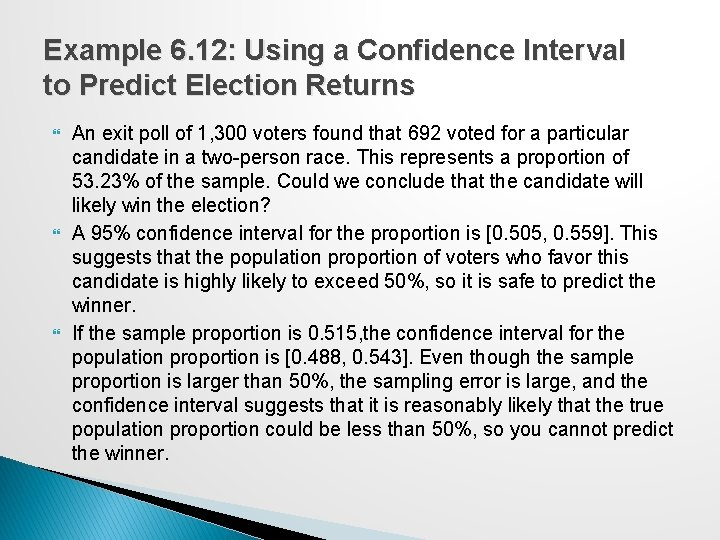Example 6. 12: Using a Confidence Interval to Predict Election Returns An exit poll of 1, 300 voters found that 692 voted for a particular candidate in a two-person race. This represents a proportion of 53. 23% of the sample. Could we conclude that the candidate will likely win the election? A 95% confidence interval for the proportion is [0. 505, 0. 559]. This suggests that the population proportion of voters who favor this candidate is highly likely to exceed 50%, so it is safe to predict the winner. If the sample proportion is 0. 515, the confidence interval for the population proportion is [0. 488, 0. 543]. Even though the sample proportion is larger than 50%, the sampling error is large, and the confidence interval suggests that it is reasonably likely that the true population proportion could be less than 50%, so you cannot predict the winner.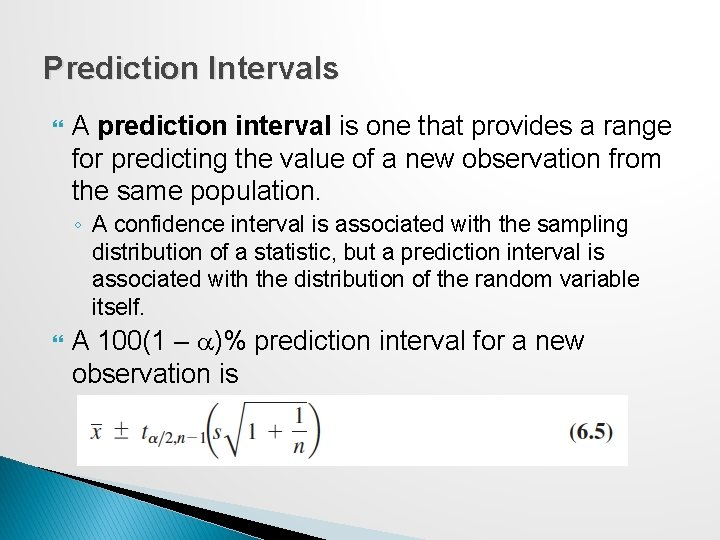Prediction Intervals A prediction interval is one that provides a range for predicting the value of a new observation from the same population. ◦ A confidence interval is associated with the sampling distribution of a statistic, but a prediction interval is associated with the distribution of the random variable itself. A 100(1 – a)% prediction interval for a new observation isExample 6. 13: Computing a Prediction Interval Compute a 95% prediction interval for the revolving balances of customers (Credit Approval Decisions)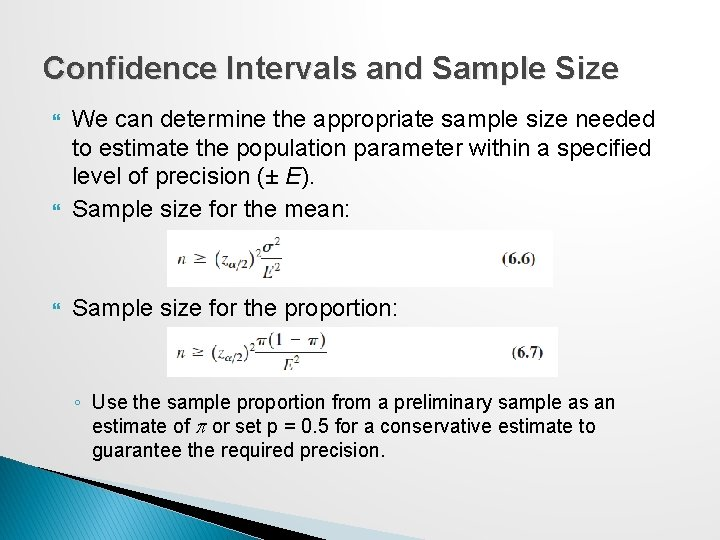Confidence Intervals and Sample Size We can determine the appropriate sample size needed to estimate the population parameter within a specified level of precision (± E). Sample size for the mean: Sample size for the proportion: ◦ Use the sample proportion from a preliminary sample as an estimate of p or set p = 0. 5 for a conservative estimate to guarantee the required precision.Example 6. 14: Sample Size Determination for the Mean In Example 6. 8, the sampling error was ± 5. 88 mls. What is sample size is needed to reduce the margin of error to at most 3 mls? Round up to 97 samples.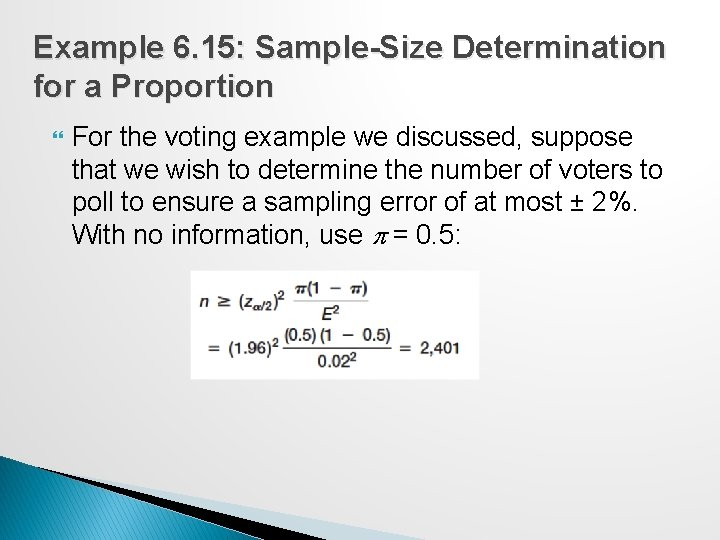Example 6. 15: Sample-Size Determination for a Proportion For the voting example we discussed, suppose that we wish to determine the number of voters to poll to ensure a sampling error of at most ± 2%. With no information, use p = 0. 5: